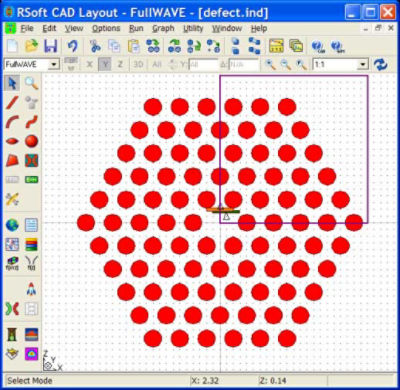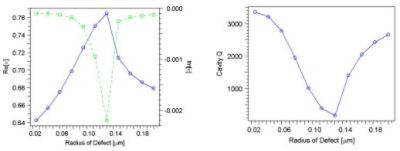# Using Q-Finder to Automate Cavity Mode and Q Calculations

## Tools Used: FullWAVE, MOST

This application example illustrates the use of Q-Finder, an automated utility for computing cavity modes and Q factors. A basic, straightforward example is provided to illustrate the use of Q-Finder, followed by a simple scanning example.

## Structure and Simulation Overview

This structure is composed of a hexagonal array of air holes in a silica slab (n=3.37). The lattice constant used is 0.4 µm along with a hole diameter of 0.65a. To create the defect, the center hole is removed. Defect modes will be looked for around a wavelength of 1.5 μm.The field is monitored within the defect region of the crystal at a position with low symmetry. This will be used by Q-Finder to compute the spectral response of the cavity. Unlike the energy decay method, which may also be used, the Fast Harmonic Analysis (FHA) method, used by Q-Finder, is able to extract accurate complex frequencies from relatively short propagation.

Several FullWAVE simulations of increasing length are performed in order to determine the resonant frequency and mode profile of the cavity mode of interest. Each simulation successively launches the mode found in the previous step in order to produce a clean mode profile. Once the simulations are completed, the final spectrum is analyzed using the FHA method to determine the Q value. This method finds a Q value of 3363 at a resonant frequency of 0.63497 μm-1, and a mode volume of 0.12.

## Basic Scanning and Optimization

Like all tools in the RSoft Photonic Device Tools, Q-Finder can be operated from within MOST to perform scanning and optimization. This example will use the same structure as above, but adds a central hole to the defect region. The size of this hole will be scanned from 0 to 0.2μm in 11 steps using MOST, and the Q will be calculated.

When the scan has finished, the results can be examined using the DataBROWSER. An interesting note is that the Q reaches a minimum at 0.13μm, which is when the defect radius matches the lattice radius effectively removing the defect and resulting in a very lossy system.